Courses

# Test: Isomerism In Coordination Compounds- II

## 25 Questions MCQ Test Chemistry Class 12 | Test: Isomerism In Coordination Compounds- II

Description
This mock test of Test: Isomerism In Coordination Compounds- II for JEE helps you for every JEE entrance exam. This contains 25 Multiple Choice Questions for JEE Test: Isomerism In Coordination Compounds- II (mcq) to study with solutions a complete question bank. The solved questions answers in this Test: Isomerism In Coordination Compounds- II quiz give you a good mix of easy questions and tough questions. JEE students definitely take this Test: Isomerism In Coordination Compounds- II exercise for a better result in the exam. You can find other Test: Isomerism In Coordination Compounds- II extra questions, long questions & short questions for JEE on EduRev as well by searching above.
QUESTION: 1

### Only One Option Correct Type Direction (Q. Nos. 1-10) This section contains 10 multiple choice questions. Each question has four choices (a), (b), (c) and (d), out of which ONLY ONE is correct. Q. Which type of isomerism is shown by [Co(NH3)4 Br2CI]?

Solution:

[Co(NH3)4Br2]CI can show both geometrical and ionisation isomerism.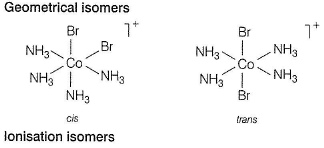[Co(NH3)4Br2]CI or [Co(NH3)4BrCI]Br

QUESTION: 2

### The complex that exists as a pair of enantiomers is

Solution:

[Co(en)3]3+, where, en = (NH2CH2CH2NH2)
The complex show optical isomerism. Optical isomers are mirror image that cannot be superimposed on one another. These are called as enantiomers. The molecules or ions that cannot be superimposed are called chiral.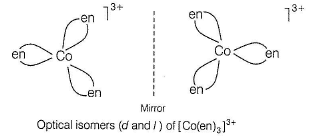QUESTION: 3

### Which of the following will have three stereoisomeric form ? i. [Cr(NO3)3(NH3)3] ii. K3[Co(C2O4)3] iii. K3[Co(C2O4)2CI2] iv. [Co(en)2CIBr]

Solution:

The complex [Co(C2O4)2Cl2]3- show both geometrical and optical isomers.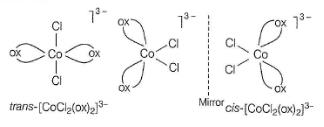QUESTION: 4

A coordination compound of cobalt has the molecular formula containing five ammonia molecules, one nitro group and two chlorine atoms for one cobalt atom. One mole of this compound produces three ions in an aqueous solution. The aqueous solution on treatment with an excess of AgNO3 gives two moles of AgCI as a precipitate. The formula of the complex and the isomerism shown by this is

Solution:

The complex [CO(NH3)5NO2]CI2  produces three ions in aqueous solution.

[Co(NH3)5NO2]CI2 → [Co(NH3)5NO2]+ 2Cl-
The aqueous solution on treatment with an excess of AgNO3 gives two moles of AgCI because we have 2 moles of Cl- ion in aqueous solution.

[Co(NH3)5NO2]CI2 and [Co(NH3)5(ONO)]CI2
The nitrite ligand is bound through oxygen (—ONO)in red form. The nitrite ligand is bound through nitrogen (—NO2) in yellow form.

QUESTION: 5

Which of the following will give maximum number of isomers?

Solution:

[Cr(SCN)2(NH3)4]+ shows linkage, geometrical and optical isomerism.

QUESTION: 6

Which of the following coordination compounds would exhibit optical isomerism?

Solution: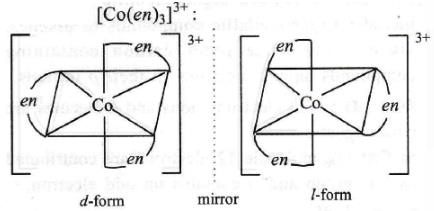QUESTION: 7

Which one of the following is expected to exhibit optical isomerism?

(en = ethylenediamine)

Solution: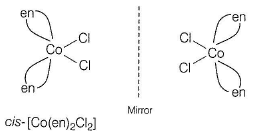QUESTION: 8

How the isomeric complexes [Co(NH3)6] [Cr(NO2)6] and [Cr(NH3)6] [Co(NO2)6] can be distinguished from one another by

Solution:

In [Co(NH3)6] [Cr(NO2)6], the [Co(NH3)6]3+ ion migrates towards the negative electrode where cobalt would be deposited. In [Cr(NH3)6] [Co(NO2)6], the [Cr(NH3)6]3+ ion migrates towards the negative electrode where chromium would be deposited.

QUESTION: 9

The complexes [Co(NH3)6] [Cr(CN)6] and [Cr(NH3)6] [Co(CN)6] are the examples of which type of isomerism?

[AIEEE 2011]

Solution:

The given complexes are the examples of coordination isomers

QUESTION: 10

The isomerism shown by [Co(EDTA)]- ion is

Solution: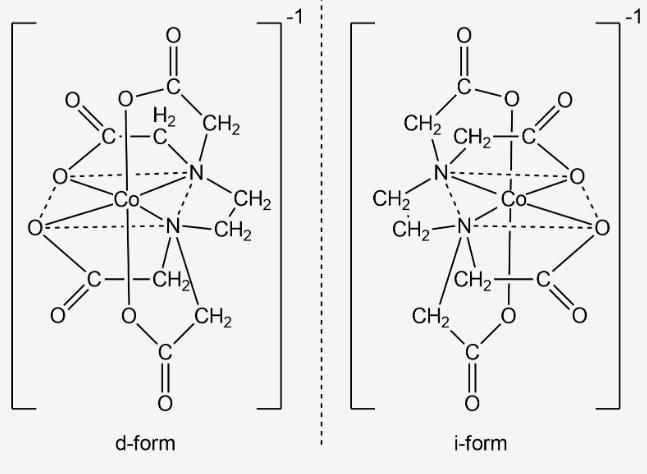*Multiple options can be correct
QUESTION: 11

Direction (Q. Nos. 11-15) This section contains 5 multiple choice questions. Each question has four choices (a), (b), (c) and (d), out of which ONE or MORE THAN ONE are correct.

Q.

[Co(NH3)4 (NO2)2]Cl exhibits

Solution:

The compound [Co(NH3)4((NO2)2]Cl exhibits linkage, ionisation and geometrical isomerism.

(i) [Co(NH3)2(NO2)2]Cl and [Co(NH3)4(ONO)2]Cl

(ii) Its ionisation isomers are
[Co(NH3)4 (NO2)Cl]NO2 and [Co(NH3)4(NO2)2]Cl

(iii) Its geometrical isomers are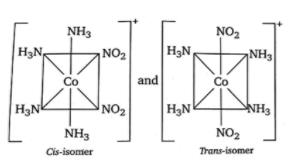*Multiple options can be correct
QUESTION: 12

The complex [Co(NH3)5Br] SO4 and [Co(NH3)5SO4] Br can be identified by

Solution:

Both given complexes can be identified by BaCI2, AgNO3 and PbCI2

*Multiple options can be correct
QUESTION: 13

The pair(s) of coordination complexes/ions exhibiting the same kind of isomerism is/are

Solution:

(a) [Cr(NH3)5CI]2+ no isomerism and [Cr(NH3)4CI2]+ show only geometrical.
(b) [Cr(NH3)4CI2]+ only geometrical and [Pt(NH3)2(H2O)CI]+ only geometrical.
(c) [CoBr2CI2]2- no isomerism because complex is tetrahedral and [ptBr2CI2]2- show geometrical.
(d) [Pt(NH3)3NO3]CI only ionisation and [Pt(NH3)3CI]Br only ionisation.

*Multiple options can be correct
QUESTION: 14

Which of the following complexes show geometrical isomerism?

Solution:

Tetrahedral complexes do not show geometrical isomerism because the relative position of the unidentate ligands attached to the central metal atoms are the same with respect to each other.

*Multiple options can be correct
QUESTION: 15

The compound(s) that exhibit(s) geometrical isomerism is/are

Solution: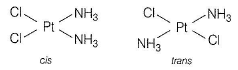Geometrical isomers (cis and trans) of [Pt(NH3)2Cl2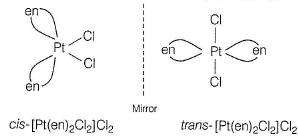QUESTION: 16

Comprehension Type

Direction (Q. Nos. 16 and 17) This section contains a paragraph, each describing theory, experiments, data, etc. Two questions related to the paragraph have been given. Each question has only one correct answer among the four given options (a), (b), (c) and (d).

Passage

A metal complex having the composition Cr(NH3)4CI2Br has been isolated in two forms A and B. The form A reacts with AgNO3 to give a white precipitate readily soluble in dilute aqueous ammonia whereas B gives a pale yellow precipitate soluble in cone, ammonia.

Q.

The formula of complex A

Solution:

[Cr(NH3)4CIBr]CI→ [Cr(NH3)4CIBr]+ + Cl-
Complex (A) give white ppt. of AgCI with AgNO3.

QUESTION: 17

A metal complex having the composition Cr(NH3)4CI2Br has been isolated in two forms A and B. The form A reacts with AgNO3 to give a white precipitate readily soluble in dilute aqueous ammonia whereas B gives a pale yellow precipitate soluble in cone, ammonia.

Q.

The isomerism shown by complex A is

Solution: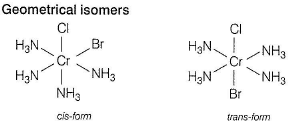Ionisation isomers [Cr(NH3)4CIBr]CI and [Cr(NH3)4CI2]Br

QUESTION: 18

Matching List Type

Direction (Q. Nos. 18 and 19) Choices for the correct combination of elements from Column I and Column II are given as options (a), (b), (c) and (d) out of which one is correct.

Q.

Match the Column I with Column II and mark the correct option from the codes given below.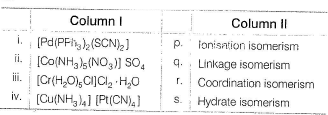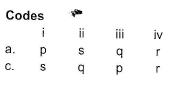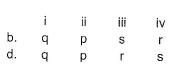Solution:

(i) → (q), (ii) → (p), (iii) → (s), (iv) → (r)

QUESTION: 19

Match the Column I with Column II and mark the correct option from the codes given below.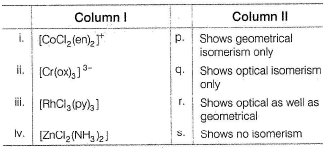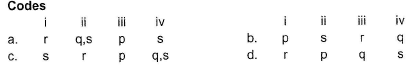Solution:

(i) → (r), (ii) → (q.s), (iii) → (p), (iv) → s

*Answer can only contain numeric values
QUESTION: 20

One Integer Value Correct Type

Direction (Q. Nos. 20 -24) This section contains 5 questions. When worked out will result in an integer from 0 to 9 (both inclusive).

Q.

The total number of isomers expected for [Pt(NCS)(en)2]2+ are

Solution:

The complex can be shown in three forms as given below: [Pt(NCS)2(en)2]2+,[Pt(SCN)2(en)2]2+ and [Pt(SCN)(NCS)(en)2]2+, Each one has a trans-isomer and dextro cis and laevo cis. Hence, total isomers are 3 x 3 = 9.

*Answer can only contain numeric values
QUESTION: 21

Number of isomers possible for [Co(NH3)5SCN]CI are

Solution:

[Co(NH3)5SCN]CI and [Co(NH3)5NCS]CI
[Co(NH3)5CI]SCN and [Co(NH3)5Cl]NCS

*Answer can only contain numeric values
QUESTION: 22

[Fe(EDTA)]- exhibits optical isomerism. In this complex, the number of rings formed are

Solution:

When[Fe(EDTA)]- exhibits optical isomerism, the complex will form five rings.

*Answer can only contain numeric values
QUESTION: 23

The number of geometrical isomers possible for the complex [CoCI2Br2]- are

Solution:

This is a tetrahedral complex.

*Answer can only contain numeric values
QUESTION: 24

The total number of possible isomers for the complex compound

[CuII(NH3)4] [PtIICI4] are

Solution:

The complex compound [Cu"(NH3)4][Pt"CI4] will form four isomers.

QUESTION: 25

Statement Type

Direction (Q. No. 25) This section is based on Statement I and Statement II. Select the correct answer from the codes given below.

Q.